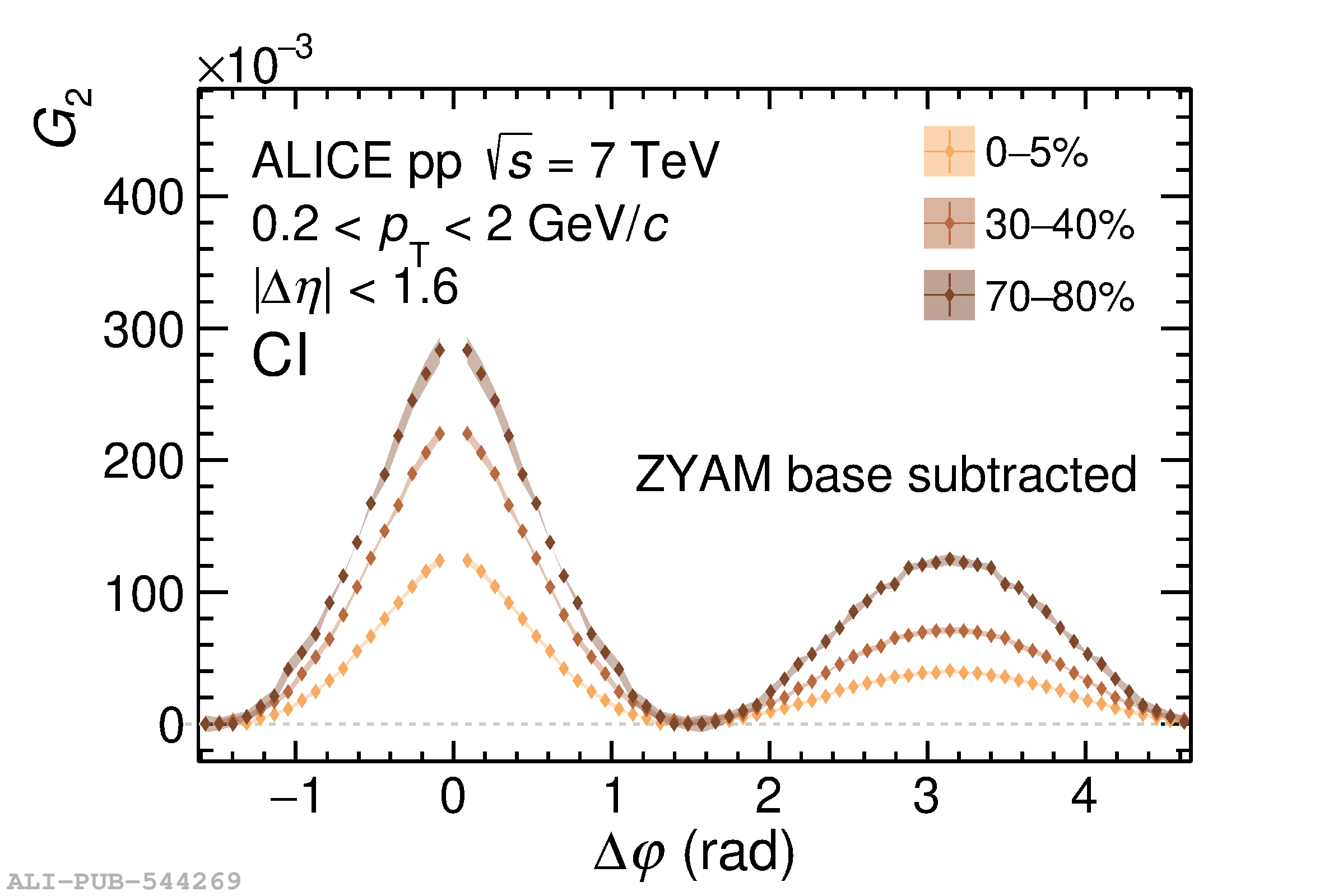# Figure 6

 Longitudinal (left) and azimuthal (right) projections of the two-particle transverse momentum correlation $G_{2}^{\rm CI}$ for selected charged particle multiplicity classes in pp collisions at $\sqrt{s}=7\;\text{TeV}$ after subtracting the azimuthal ZYAM base level (see text for details) for each multiplicity class. Vertical bars (mostly smaller than the marker size) and shaded bands represent statistical and uncorrelated systematic uncertainties, respectively.